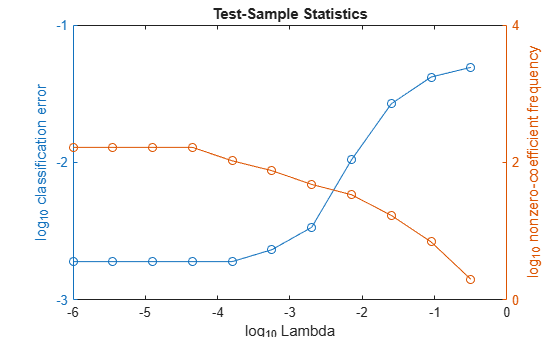selectModels

Class: ClassificationLinear

Choose subset of regularized, binary linear classification models

Description

example

SubMdl = selectModels(Mdl,idx) returns a subset of trained, binary linear classification models from a set of binary linear classification models (Mdl) trained using various regularization strengths. The indices (idx) correspond to the regularization strengths in Mdl.Lambda, and specify which models to return.

Input Arguments

expand all

Binary linear classification models trained using various regularization strengths, specified as a ClassificationLinear model object. You can create a ClassificationLinear model object using fitclinear.

Although Mdl is one model object, if numel(Mdl.Lambda) = L ≥ 2, then you can think of Mdl as L trained models.

Indices corresponding to regularization strengths, specified as a numeric vector of positive integers. Values of idx must be in the interval [1,L], where L = numel(Mdl.Lambda).

Data Types: double | single

Output Arguments

expand all

Subset of binary linear classification models trained using various regularization strengths, returned as a ClassificationLinear model object.

Examples

expand all

To determine a good lasso-penalty strength for a linear classification model that uses a logistic regression learner, compare test-sample classification error rates.

Load the NLP data set. Preprocess the data as in Specify Custom Classification Loss.

Ystats = Y == 'stats';
X = X';

rng(10); % For reproducibility
Partition = cvpartition(Ystats,'Holdout',0.30);
testIdx = test(Partition);
XTest = X(:,testIdx);
YTest = Ystats(testIdx);

Create a set of 11 logarithmically-spaced regularization strengths from $1{0}^{-6}$ through $1{0}^{-0.5}$.

Lambda = logspace(-6,-0.5,11);

Train binary, linear classification models that use each of the regularization strengths. Optimize the objective function using SpaRSA. Lower the tolerance on the gradient of the objective function to 1e-8.

CVMdl = fitclinear(X,Ystats,'ObservationsIn','columns',...
'CVPartition',Partition,'Learner','logistic','Solver','sparsa',...
CVMdl =
ClassificationPartitionedLinear
CrossValidatedModel: 'Linear'
ResponseName: 'Y'
NumObservations: 31572
KFold: 1
Partition: [1x1 cvpartition]
ClassNames: [0 1]
ScoreTransform: 'none'

Properties, Methods

Extract the trained linear classification model.

Mdl = CVMdl.Trained{1}
Mdl =
ClassificationLinear
ResponseName: 'Y'
ClassNames: [0 1]
ScoreTransform: 'logit'
Beta: [34023x11 double]
Bias: [-12.0872 -12.0872 -12.0872 -12.0872 -12.0872 ... ]
Lambda: [1.0000e-06 3.5481e-06 1.2589e-05 4.4668e-05 ... ]
Learner: 'logistic'

Properties, Methods

Mdl is a ClassificationLinear model object. Because Lambda is a sequence of regularization strengths, you can think of Mdl as 11 models, one for each regularization strength in Lambda.

Estimate the test-sample classification error.

ce = loss(Mdl,X(:,testIdx),Ystats(testIdx),'ObservationsIn','columns');

Because there are 11 regularization strengths, ce is a 1-by-11 vector of classification error rates.

Higher values of Lambda lead to predictor variable sparsity, which is a good quality of a classifier. For each regularization strength, train a linear classification model using the entire data set and the same options as when you cross-validated the models. Determine the number of nonzero coefficients per model.

Mdl = fitclinear(X,Ystats,'ObservationsIn','columns',...
'Learner','logistic','Solver','sparsa','Regularization','lasso',...
numNZCoeff = sum(Mdl.Beta~=0);

In the same figure, plot the test-sample error rates and frequency of nonzero coefficients for each regularization strength. Plot all variables on the log scale.

figure;
[h,hL1,hL2] = plotyy(log10(Lambda),log10(ce),...
log10(Lambda),log10(numNZCoeff + 1));
hL1.Marker = 'o';
hL2.Marker = 'o';
ylabel(h(1),'log_{10} classification error')
ylabel(h(2),'log_{10} nonzero-coefficient frequency')
xlabel('log_{10} Lambda')
title('Test-Sample Statistics')
hold offChoose the index of the regularization strength that balances predictor variable sparsity and low classification error. In this case, a value between $1{0}^{-4}$ to $1{0}^{-1}$ should suffice.

idxFinal = 7;

Select the model from Mdl with the chosen regularization strength.

MdlFinal = selectModels(Mdl,idxFinal);

MdlFinal is a ClassificationLinear model containing one regularization strength. To estimate labels for new observations, pass MdlFinal and the new data to predict.

Tips

One way to build several predictive, binary linear classification models is:

1. Hold out a portion of the data for testing.

2. Train a binary, linear classification model using fitclinear. Specify a grid of regularization strengths using the 'Lambda' name-value pair argument and supply the training data. fitclinear returns one ClassificationLinear model object, but it contains a model for each regularization strength.

3. To determine the quality of each regularized model, pass the returned model object and the held-out data to, for example, loss.

4. Identify the indices (idx) of a satisfactory subset of regularized models, and then pass the returned model and the indices to selectModels. selectModels returns one ClassificationLinear model object, but it contains numel(idx) regularized models.

5. To predict class labels for new data, pass the data and the subset of regularized models to predict.